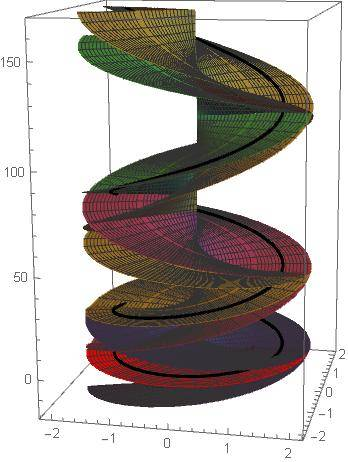# Analytic continuation of Dilogarithm

• I
aheight
Summary
I cannot understand the notation for analytically-continuing this function as given by Lewen and was wondering if someone could help me.
I am trying to understand the branching geometry of the Dilogarithm function as described in Branching geometry of Dilogarithm. In Theorem 8.6, the following (dilogarithm) definition is given by letting ##n=2##:

$$\text{Li}_2(z)=\text{Li}_2^{(k_0,k_1)}(z)=\text{Li}_2^{(0)}(z)+\sum_{m=0}^1 k_m\binom{1}{m}(2\pi i)^{m+1}(\text{Log}(z))^{2-m-1}$$
but it's not clear at all what ##k_m## is and was wondering if someone would know this?

For example, suppose I wanted to plot an analytically-continuous ##6\pi## route via ##z(t)=2e^{it}## over it's Riemann surface, how would I splice-together the pieces defined above over the branch-cuts ##(-\infty,0)## and ##(1,\infty)## beginning on the principal branch ##\text{Li}_2(2)## so that the path traverses an analytically-continuous over them?

I can differentially-continue the path above over the sheets of ##f(z)=\text{Li}_2(z)## via the IVP:
$$\frac{df}{dt}=-\frac{1}{2}\log(1-z)\frac{dz}{dt};\quad f(0)=\text{Li}_2(2)$$
and by using MapleSoft's definition:

Start with the principal sheet ##\text{Li}_n## (implemented in mathematica as ##\text{Polylog}[n,z]##. Now, each time the branch cut ##(1,\infty)## is crossed in the counter clockwise direction, subtract from the current expression ##\frac{2\pi i\text{Log}^{n-1}(z)}{\Gamma(n)}##. Each time the branch cut ##(-\infty,0)## is crossed in the counter clockwise direction, add ##2\pi i## to the ##\text{Log}## term.

stitch the MapleSoft sheets together by trial-and-error over this path to create the (real) plot below showing the analytically-continuous route through the function along this path in black. However that's not practical and was hoping to better understand the formula for ##\text{Li}_2(z)## above to do this systematically by supplying the correct indices ##k_m## I assume.Last edited:

Mentor
Well, it looks like there are only two ##k_m## variables namely: ##k_0## and ##k_1##.

I think they are arbitrarily set values to cover a family of such surfaces.

Is there nothing that defines or limits the values of the ##k_m## in the book or theorem statement?

NOTE: ALso it looks like your Maplesoft link no longer works. It sends me to a new Google Books web page with no means to see the pages you've referenced.

aheight
NOTE: ALso it looks like your Maplesoft link no longer works. It sends me to a new Google Books web page with no means to see the pages you've referenced.

Is there nothing that defines or limits the values of the km in the book or theorem statement?

Not that I can see.

Last edited:
Mentor
Okay got it. I had to press the preview button to see it.

On page 175 they mention the k values are computed as follows:

##(1+k)^0 = k_0##

##(1+k)^m = k_0 + \binom{m}{1} k_1 + ... + \binom{m}{n} k_m ##

aheight
Okay got it. I had to press the preview button to see it.

On page 175 they mention the k values are computed as follows:

##(1+k)^0 = k_0##

##(1+k)^m = k_0 + \binom{m}{1} k_1 + ... + \binom{m}{n} k_m ##

I did see that but what is ##k## then in regards to the summation?

Mentor
On page 174, they mention the integral of order k-1 and then consider the integral of k=1 so k apparently is an integer value ranging from k=1 to k=n?

This may be off-base but could it be like differential forms of order k notion?

https://en.wikipedia.org/wiki/Differential_form

A differential k-form can be integrated over an oriented k-dimensional manifold. When the k-form is defined on an n-dimensional manifold with n > k, then the k-form can be integrated over oriented k-dimensional submanifolds. If k = 0, integration over oriented 0-dimensional submanifolds is just the summation of the integrand evaluated at points, with according to the orientation of those points. Other values of k = 1, 2, 3, ... correspond to line integrals, surface integrals, volume integrals, and so on. There are several equivalent ways to formally define the integral of a differential form, all of which depend on reducing to the case of Euclidean space.

aheight
On page 174, they mention the integral of order k-1 and then consider the integral of k=1 so k apparently is an integer value ranging from k=1 to k=n?

Ok thanks. It's a bit tough for me to follow and I don't know differential forms. I could try letting k=0 or 1 but still not clear to me how to systematically link the branching across the branch-cuts as shown in Figure 8.2.

The reason I'm asking is because my experimental results via the IVP above do not agree with the MapleSoft definition of incrementing by 2πi (or Lewen's apparently) each time the branch cut (−∞,0) is crossed. In the plot I showed above, I needed to increment some of the branching by just πi to obtain an analytically-continuous route through the function so I wanted to know what is causing this discrepancy.

aheight
Just a correction to the above:

In my initial post above I described differential-continuation of the Dilogarithm function. However, it was poorly described and I wish to better do so here so that that interested reader can understand the principle when the DE of a multi-valued function contains itself, a multi-valued function: First continue the contained multi-valued function, then use that version in the original DE. Below, first continue ##\log(1-z)##, then use it in the Dilogarithm IVP to subsequently continue Dilogarithm:

Letting ##z(t)=1.5 e^{i t}## with ##0\leq t\leq 6\pi ##, ##u(z)=\log(1-z)## and ##f(z)=\text{Li}_2(z)##, first continue ##u(z(t))## then use ##u(t)## in the IVP for the Dilogarithm. That is, in this particular example, starting on the principal-valued log sheet, numerically solve sequentially,
\begin{align*} \frac{du}{dt}&=-\frac{1}{1-z(t)}\frac{dz}{dt};\quad u(0)=\text{Log}(1-z(0)) \\ \frac{df}{dt}&=-\frac{1}{z(t)}u(t)\frac{dz}{dt};\quad f(0)=\text{Li}_2(1.5) \end{align*}

This is how the (Re) black contour along the sheets of Dilogarithm in the plot above was generated.

Last edited: